•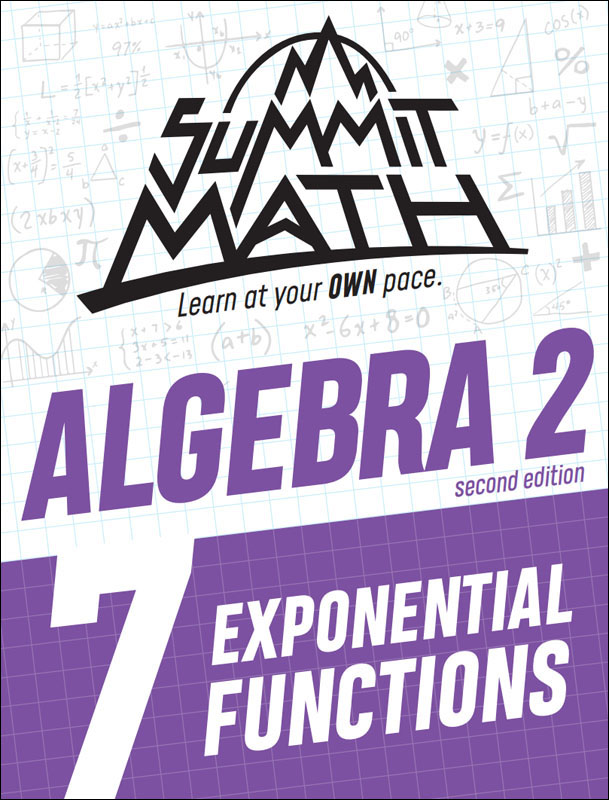•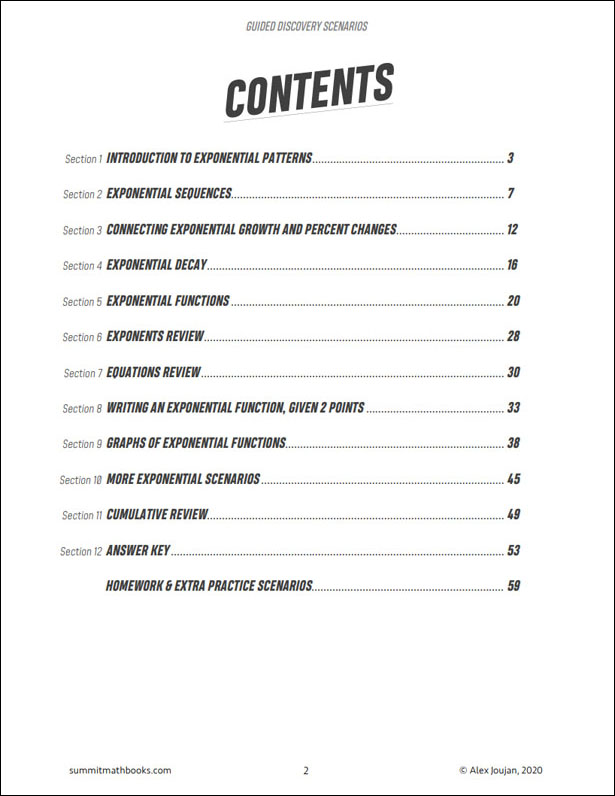•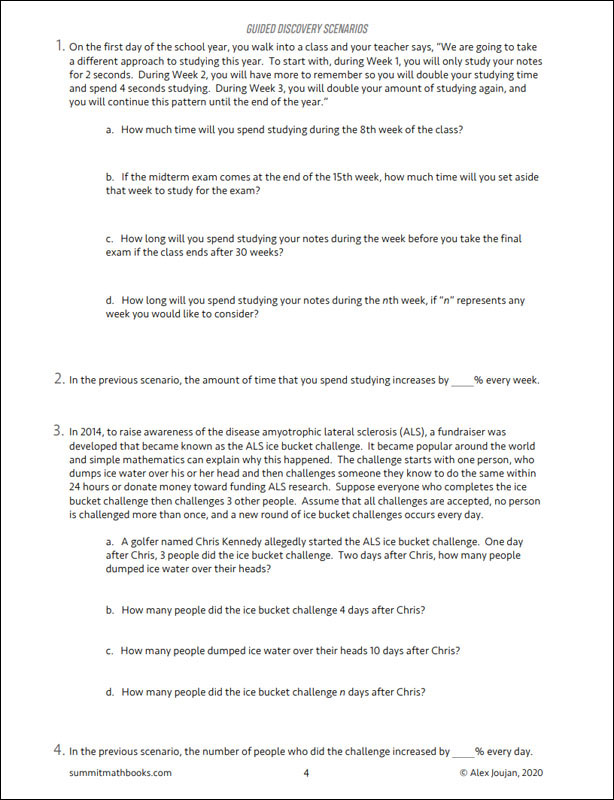•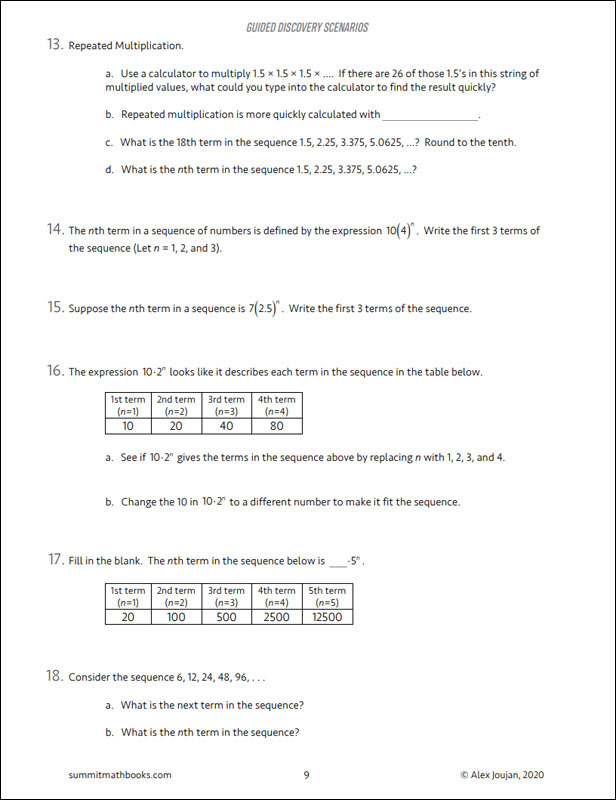•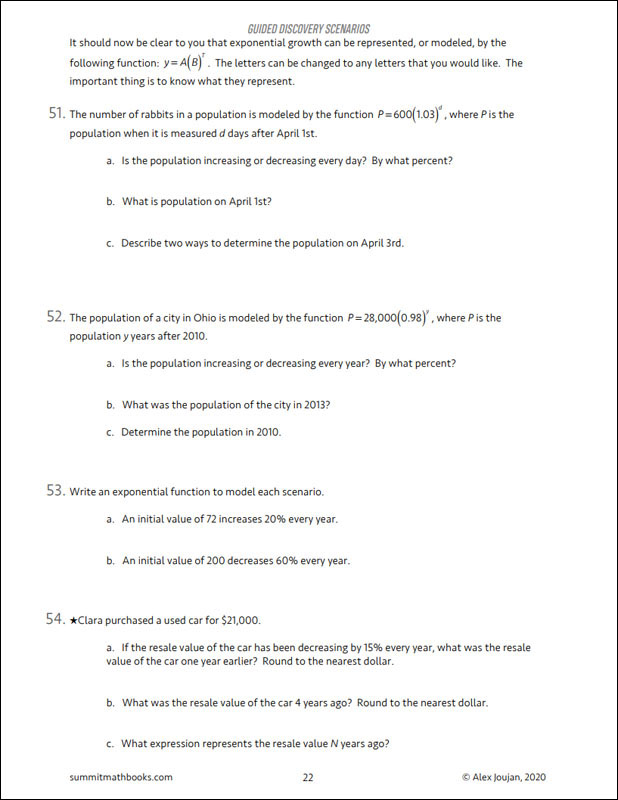# Summit Math Algebra 2 Book 7: Exponential Functions (2nd Edition)

# 078926

Our Price: \$12.25
Retail: \$12.50
Save: 2.00% (\$0.25)
In Stock.
Qty:
Qty:

Item #: 078926 9781712190111 7-11

#### Publisher Description:

In this book, students learn that exponential patterns come from repeated multiplication. They also learn that exponential patterns can be viewed as repeated percentage changes. They investigate scenarios that involve both exponential growth and decay. They learn how to graph exponential functions. They also learn how to use systems of equations to find the equation for an exponential function when they know 2 points. This book builds on Algebra 1: Book 1 and Algebra 2: Book 6.

Topics in this book:

• Introduction to exponential patterns
• Exponential sequences
• Connecting exponential growth and percent changes
• Exponential decay
• Exponential functions
• Exponents review
• Equations review
• Writing an exponential function, given 2 points
• Graphs of exponential functions
• More exponential scenarios
• Cumulative review

Category Description for Summit Math Algebra:

If you are searching for an independent algebra program or a supplement to teach specific algebra concepts, Summit Math is worth your consideration. Using the guided discovery method, students make their own connections as they learn to master each scenario before moving on to the next. Each section builds on previous concepts already learned. The first half of the book incorporates the guided discovery approach, while the latter half uses extra practice sheets to improve understanding of the topics. Each section has an answer key. A placement test and pacing guide is provided for each level. The author states this is a complete program teaching typical topics in the Algebra I and II sequence while preparing students for standardized testing. If students feel they need extra instruction, a video subscription is available from the publisher. Free downloadable tests and a more detailed version of the answer key are also available at the publisher’s website.

Primary Subject
Mathematics
7
11
ISBN
9781712190111
Format
Paperback
Brand Name
Summit Math
Weight
0.75 (lbs.)
Dimensions
10.94" x 8.5" x 0.31"